NEET  >  31 Year NEET Previous Year Questions: Some Basic Concepts Of Chemistry - 1

# 31 Year NEET Previous Year Questions: Some Basic Concepts Of Chemistry - 1 - NEET

Test Description

## 20 Questions MCQ Test Chemistry Class 11 - 31 Year NEET Previous Year Questions: Some Basic Concepts Of Chemistry - 1

31 Year NEET Previous Year Questions: Some Basic Concepts Of Chemistry - 1 for NEET 2023 is part of Chemistry Class 11 preparation. The 31 Year NEET Previous Year Questions: Some Basic Concepts Of Chemistry - 1 questions and answers have been prepared according to the NEET exam syllabus.The 31 Year NEET Previous Year Questions: Some Basic Concepts Of Chemistry - 1 MCQs are made for NEET 2023 Exam. Find important definitions, questions, notes, meanings, examples, exercises, MCQs and online tests for 31 Year NEET Previous Year Questions: Some Basic Concepts Of Chemistry - 1 below.
Solutions of 31 Year NEET Previous Year Questions: Some Basic Concepts Of Chemistry - 1 questions in English are available as part of our Chemistry Class 11 for NEET & 31 Year NEET Previous Year Questions: Some Basic Concepts Of Chemistry - 1 solutions in Hindi for Chemistry Class 11 course. Download more important topics, notes, lectures and mock test series for NEET Exam by signing up for free. Attempt 31 Year NEET Previous Year Questions: Some Basic Concepts Of Chemistry - 1 | 20 questions in 20 minutes | Mock test for NEET preparation | Free important questions MCQ to study Chemistry Class 11 for NEET Exam | Download free PDF with solutions
 1 Crore+ students have signed up on EduRev. Have you?
31 Year NEET Previous Year Questions: Some Basic Concepts Of Chemistry - 1 - Question 1

### An organic compound contains 80% (by wt.) carbon and the remaining percentage of hydrogen. The right option for the empirical formula of this compound is: [Atomic wt. of C is 12, H is 1]     (2021)

Detailed Solution for 31 Year NEET Previous Year Questions: Some Basic Concepts Of Chemistry - 1 - Question 1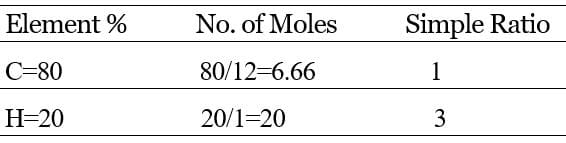Hence empirical formula =CH3

Additional Information: An empirical formula represents the simplest whole-number ratio of various atoms present in a compound, whereas, the molecular formula shows the exact number of different types of atoms present in a molecule of a compound.

31 Year NEET Previous Year Questions: Some Basic Concepts Of Chemistry - 1 - Question 2

### Which one of the followings has maximum number of atoms?     (2020)

Detailed Solution for 31 Year NEET Previous Year Questions: Some Basic Concepts Of Chemistry - 1 - Question 2

We know that,

Number of atoms =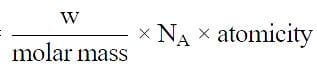(a) no. of atoms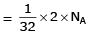(b) no. of atoms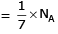(c) no. of atoms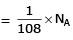(d) no. of atoms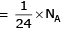∴ 1 gm of Li is having maximum no. of atoms.

31 Year NEET Previous Year Questions: Some Basic Concepts Of Chemistry - 1 - Question 3

### In an experiment it showed that 10 mL of 0.05 M solution of chloride required 10 mL of 0.1 M solution of AgNO3, which of the following will be the formula of the chloride (X stands for the symbol of the element other than chlorine): [NEET Kar. 2013]

Detailed Solution for 31 Year NEET Previous Year Questions: Some Basic Concepts Of Chemistry - 1 - Question 3

Millimoles of solution of chloride = 0.05 × 10 = 0.5
Millimoles of AgNO3 solution = 10 × 0.1 = 1
So, the millimoles of AgNO3 are double than the chloride solution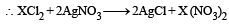31 Year NEET Previous Year Questions: Some Basic Concepts Of Chemistry - 1 - Question 4

6.02 × 1020 molecules of urea are present in 100 mL of its solution. The concentration of solution is: [NEET 2013]

Detailed Solution for 31 Year NEET Previous Year Questions: Some Basic Concepts Of Chemistry - 1 - Question 4

Given, the number of molecules of urea =6.02 x 1020
therefore, the number of moles =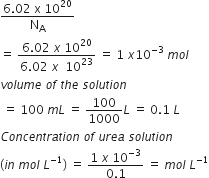Therefore, the correct answer is 0.01 M

31 Year NEET Previous Year Questions: Some Basic Concepts Of Chemistry - 1 - Question 5

Which has the maximum number of molecules among the following ? [2011 M]

Detailed Solution for 31 Year NEET Previous Year Questions: Some Basic Concepts Of Chemistry - 1 - Question 5
• The number of moles can be calculated from the ratio of the mass to the molar mass.
• A maximum number of moles will correspond to the maximum number of molecules.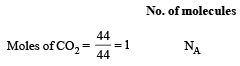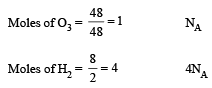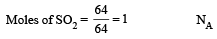Hence, option C is correct.

31 Year NEET Previous Year Questions: Some Basic Concepts Of Chemistry - 1 - Question 6

The number of atoms in 0.1 mole of a triatomic gas is:  (NA = 6.02 ×1023 mol–1)

Detailed Solution for 31 Year NEET Previous Year Questions: Some Basic Concepts Of Chemistry - 1 - Question 6

1 mol of triatomic gas has 3 ×6.02×1023 atoms

Therefore, no. of atoms in 0.1 mol =0.1×  3 ×6.02×1023 = 1.806×1023

31 Year NEET Previous Year Questions: Some Basic Concepts Of Chemistry - 1 - Question 7

What is the [OH] in the final solution prepared by mixing 20.0 mL of 0.050 M HCl with 30.0 mL of 0.10 M Ba(OH)2? 

Detailed Solution for 31 Year NEET Previous Year Questions: Some Basic Concepts Of Chemistry - 1 - Question 7

Ba(OH)2​+2HCl⟶BaCl2​+2H2​O

No. of milli equivalent of HCl = 20 × 0.05 = 1.0
No. of milli equivalent of Br (OH)2   = 30 × 0.1 × 2 = 6.0
After neutralization, no. of milli equivalents in 50 ml. of solution = (6 – 1) = 5
Total volume of the solution = 20 + 30 = 50 ml
∴ No. of milli equivalent of OHis 5 in 50 ml,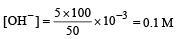31 Year NEET Previous Year Questions: Some Basic Concepts Of Chemistry - 1 - Question 8

How many moles of lead (II) chloride will be formed from a reaction between 6.5 g of PbO and 3.2 g of HCl ? 

Detailed Solution for 31 Year NEET Previous Year Questions: Some Basic Concepts Of Chemistry - 1 - Question 8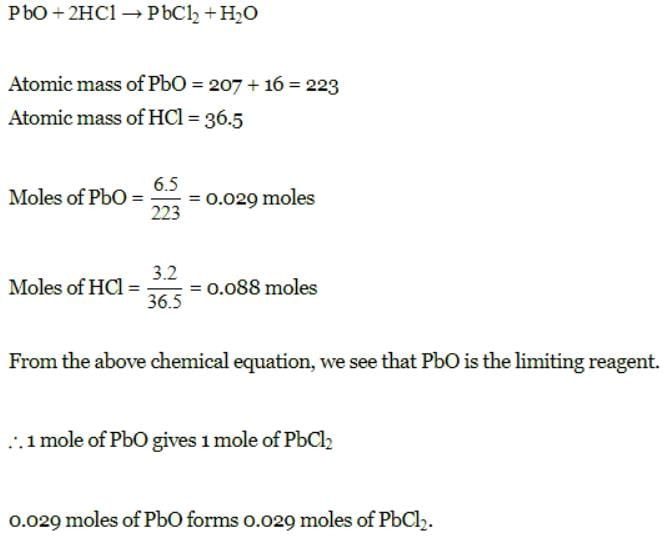Therefore, the correct answer is D.

31 Year NEET Previous Year Questions: Some Basic Concepts Of Chemistry - 1 - Question 9

An organic compound contains carbon, hydrogen and oxygen. Its elemental analysis gave C, 38.71% and H, 9.67%. The empirical formula of the compound would be: 

Detailed Solution for 31 Year NEET Previous Year Questions: Some Basic Concepts Of Chemistry - 1 - Question 9

The empirical formula is to be calculated as:Thus empirical formula is CH3O.

31 Year NEET Previous Year Questions: Some Basic Concepts Of Chemistry - 1 - Question 10

What volume of oxygen gas (O2) measured at 0°C and 1 atm, is needed to burn completely 1L of propane gas (C3H8) measured under the same conditions? 

Detailed Solution for 31 Year NEET Previous Year Questions: Some Basic Concepts Of Chemistry - 1 - Question 10

Writing the equation of  combustion of propane (C3H8), we get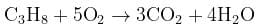From the above equation we find that we need 5 L of oxygen at NTP to completely burn 1 L of propane at N.T.P.
If we change the conditions for both the gases from N.T.P. to same conditions of temperature and pressure. The same results are obtained. i.e. 5 L is the correct answer.

31 Year NEET Previous Year Questions: Some Basic Concepts Of Chemistry - 1 - Question 11

Number of moles of MnO4- required to oxidize one mole of ferrous oxalate completely in acidic medium will be : 

Detailed Solution for 31 Year NEET Previous Year Questions: Some Basic Concepts Of Chemistry - 1 - Question 11

No. of moles of MnO4−  required to oxidize one mole of FeC2​O4​ completely in an acidic medium.

3MnO4− ​+ 24H+ 5FeC2​O4​⟶ 3Mn+2 + 12H2​O + 10CO2 ​+ 5Fe+3

5 moles of  FeC2​O4 reacts with 3 moles of MnO4−.

5 moles of FeC2​O4​⟶3 moles of MnO4−

1 mole of FeC2​O4​⟶ 53​ moles of MnO4−​

∴ The number of moles of MnO4−​ required to oxidize one mole of ferrous oxalate completely in the acidic medium will be 0.6 moles.

31 Year NEET Previous Year Questions: Some Basic Concepts Of Chemistry - 1 - Question 12

Volume occupied by one molecule of water (density = 1 g cm–3) is : 

Detailed Solution for 31 Year NEET Previous Year Questions: Some Basic Concepts Of Chemistry - 1 - Question 12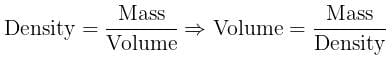Density of water = 1 gram cm–3
∴ Volume occupied by 1 gram water = 1 cm3
i.e. Volume occupied by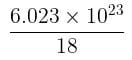molecules of water = 1 cm3 [∵ 1g water = 1/18moles of water]

Thus volume occupied by 1 molecule of water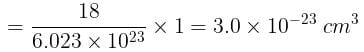31 Year NEET Previous Year Questions: Some Basic Concepts Of Chemistry - 1 - Question 13

The number of moles of KMnO4 that will be needed to react with one mole of sulphite ion in acidic solution is 

Detailed Solution for 31 Year NEET Previous Year Questions: Some Basic Concepts Of Chemistry - 1 - Question 13

The balanced chemical equation is: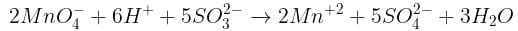From the equation, it is clear that 2 moles of MnO4  are required to oxidize 5 moles of SO3–2

2/5 mole of MnO4 is required to oxidize 1 mole of SO3–2

31 Year NEET Previous Year Questions: Some Basic Concepts Of Chemistry - 1 - Question 14

Concentrated aqueous sulphuric acid is 98% H2SO4 by mass and has a density of 1.80 g mL– 1. Volume of acid required to make one litre of 0.1MH2SO4 solution is 

Detailed Solution for 31 Year NEET Previous Year Questions: Some Basic Concepts Of Chemistry - 1 - Question 14

Molarity of H2SO4 solution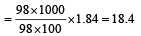Suppose V ml of this H2SO4 is used to prepare 1 lit. of 0.1M H2SO4
∴ V x 18.02 = 1000 x 0.1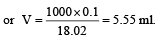31 Year NEET Previous Year Questions: Some Basic Concepts Of Chemistry - 1 - Question 15

The number of moles of KMnO4 reduced by one mole of KI in alkaline medium is:   

Detailed Solution for 31 Year NEET Previous Year Questions: Some Basic Concepts Of Chemistry - 1 - Question 15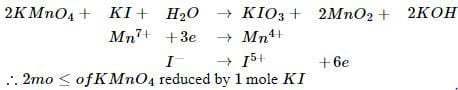31 Year NEET Previous Year Questions: Some Basic Concepts Of Chemistry - 1 - Question 16

The mass of carbon anode consumed (givin g only carbondioxide) in the production of 270 kg of aluminium metal from bauxite by the Hall process is  (Atomic mass: Al = 27) 

Detailed Solution for 31 Year NEET Previous Year Questions: Some Basic Concepts Of Chemistry - 1 - Question 16

2Al2O3 + 3C → Al3CO2

Gram equivalent  of Al2O3 = Gram equivalent of C

Equivalent weight of Al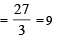Equivalent weight of C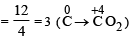No. of gram equivalent of Al  =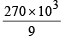= 30 × 103
Hence, No. of gram equivalent of C = 30 × 103

Again, No. of gram equivalent of C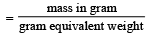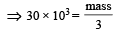⇒  mass = 90 × 10g = 90 kg

31 Year NEET Previous Year Questions: Some Basic Concepts Of Chemistry - 1 - Question 17

In Haber process 30 litres of dihydrogen and 30 litres of dinitrogen were taken for reaction which yielded only 50% of the expected product. What will be the composition of gaseous mixture under the aforesaid condition in the end? 

Detailed Solution for 31 Year NEET Previous Year Questions: Some Basic Concepts Of Chemistry - 1 - Question 17It is given that only 50% of the expected product is formed.
Hence, only 10 litre of NH3 is formed i.e. N2 used = 5 litres ⇒ N2 left = 30 – 5 = 25 litres
H2 used = 15 litres ⇒ H2 left = 30 – 15 = 15 litres

31 Year NEET Previous Year Questions: Some Basic Concepts Of Chemistry - 1 - Question 18

The maximum number of molecules is present in:

Detailed Solution for 31 Year NEET Previous Year Questions: Some Basic Concepts Of Chemistry - 1 - Question 18

As we know, 1 mole of gas has NA​ molecules. So, the higher the number of moles of a gas, it will have higher the number of molecules.

No. of molecules in different cases:

(a) 22.4 litre at STP contains = 6.023×1023 molecule of H2
∴ 15 litre at STP contains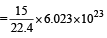molecules

(b) 22.4 litre at STP contains = 6.023×1023 molecule of N2
∴ 5 litre at STP contains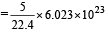molecules

(c) 2 gm of  H= 6.023×1023 molecules of  H2
∴ 0.5 gm of H2 contains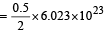molecules

(d) Similarly, 10 g of O2 gas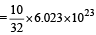molecules

Thus (a) will have maximum number of molecules

31 Year NEET Previous Year Questions: Some Basic Concepts Of Chemistry - 1 - Question 19

An element , X has the following isotopic composition :
200X:90 %
199X : 8.0%
202X ; 2.0 %
The weighted average atomic mass of the naturally occuring element X is closest to

Detailed Solution for 31 Year NEET Previous Year Questions: Some Basic Concepts Of Chemistry - 1 - Question 19

Average isotopic mass of,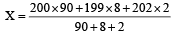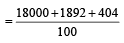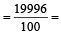= 199.96 amu ≈200 amu

31 Year NEET Previous Year Questions: Some Basic Concepts Of Chemistry - 1 - Question 20

10 g of hydrogen and 64 g of oxygen were filled in a steel vessel and exploded. Amount of water produced in this reaction will be:

Detailed Solution for 31 Year NEET Previous Year Questions: Some Basic Concepts Of Chemistry - 1 - Question 20

2H​+ O2​ → 2H2​O

10 g of hydrogen (molar mass 2 g/mol) = 2 g/mol  10 g ​= 5 mol

64 of oxygen  (molar mass 32 g/mol) = 32 g/mol  64 g ​= 2 mol

As per the balanced chemical equation, 1 mole of oxygen will react with 2 moles of hydrogen to form 2 moles of water.

Here, oxygen is the limiting reagent and hydrogen is the excess reagent.

Hence the amount of H2O produced depends on the amount of Otaken
0.5 mole of O2 gives H2O = 1 mol
∴ 2 mole of O2 gives H2O = 4 mol

## Chemistry Class 11

195 videos|336 docs|190 tests
Information about 31 Year NEET Previous Year Questions: Some Basic Concepts Of Chemistry - 1 Page
In this test you can find the Exam questions for 31 Year NEET Previous Year Questions: Some Basic Concepts Of Chemistry - 1 solved & explained in the simplest way possible. Besides giving Questions and answers for 31 Year NEET Previous Year Questions: Some Basic Concepts Of Chemistry - 1, EduRev gives you an ample number of Online tests for practice

## Chemistry Class 11

195 videos|336 docs|190 tests

### How to Prepare for NEET

Read our guide to prepare for NEET which is created by Toppers & the best Teachers